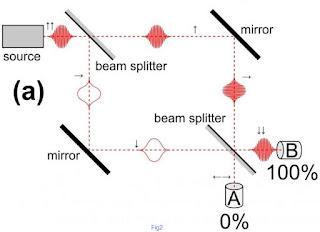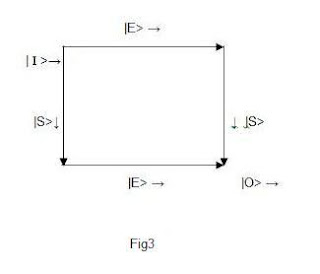## Monday, May 20, 2013

### Mach–Zehnder interferometer Particle or Wave?

This is a continuation of what we have covered in the two-slit experiment. If you have forgotten the main concepts, kindly review them.

We will try to shed some light on what makes up light: particles or waves.

In papers written about this subject, such as of the Mach-Zehnder interferometer, you will often read: "The explanation for this result is that it appears a single photon travels both paths and engages in interference with itself." Language fails us when we are trying to describe a QM system. However, the language of mathematics can compensate.

So first, a few words on the devise in question we will use for this blog. The Mach-Zehnder interferometer is made of a collimated beam which is split by a half-silvered mirror or a beam splitter. The two resulting beams are each reflected by a mirror. The two beams then pass a second beam splitter and enter a number of detectors - in figure 1(a),(b), there are two detectors A and B; in figure 1(c) there three detectors, C being the additional one.Fig 1

The enigma is in figure 1(a). So before we tackle this situation, we will look at figure 1(c), reproduced below.Fig 1(C)

To keep track of the different beams, we use E, for beaming going East, S for south, N for north, etc. The incoming beam is labelled I, the outgoing one, O. (See figure 3, below)

After the incoming wave goes through the split beam, (top left-hand corner) we have.

| I > → |E> + |S>

The East going state will reflect at the mirror and then go South, to undergo another split at the bottom right-hand corner, and then go the detector A and B. We can write this whole sequence as,

|E> → |S> → 2-1/2(a|A> + b|B>)

Here the constants a and b are needed as we must normalize the state vector. This is another reason why we shouldn't take the wavefunction in QM as real. A better language is the state vector using the Dirac notation as we are doing. We normalize it because the state vectors in QM allow us to calculate probabilities, and a simple mathematical rule is that the sum of all probabilities in a given situation adds up to 1. So we need to put another constant for the South going state, which will be reflected by the bottom left-hand mirror, to go Eastward.

|S> → |E> → c|C>

Therefore, | I > = 2-1/2(a|A> + b|B>) + c|C>

Normalization

< I | I > = a2/2 + b2/2 + c2 = 1

By symmetry, we can choose: a = b

a2 + c2 = 1

Giving us a = b = c = 2-1/2

Putting this altogether:

| I > = 2-1|A> + 2-1|B> + 2-1/2|C>

A little calculation will give:

Probability at A is the square of the amplitude:

P(A) = │< I | A >│2 = 1/4.

Similarly, P(B) = 1/4 and P(C) = 1/2

Particle or WaveFig 2

At the heart of the mystery - particle or wave - is what's happening in figure 1(a), reproduced above in figure 2. Keep in mind that the light intensity has been reduced so that only one photon at a time travels through the apparatus. What is seemingly unexplanable are the numbers, which read as: 0% in detector A, and 100% in detector B. Even if the single photon would interfer constructively with itself at the beam-splitter(bottom right-hand corner), after that, each wave should continue along their path, and 50% should go to A, 50% to B. But we don't get that, instead we get 100% at B, none at A. That would mean the wave coming from the southern branch moving from left to right, goes 100% (of 50%)through the beam-splitter(bottom right-hand corner), IOW, doesn't split and continues to B (depicted in fig 1b), while the northern branch, which is now moving downward after reflection with the mirror(top right-hand corner), but now makes an extraordinary 90 degree turn at the beam-splitter (bottom right-hand corner), without splitting to move 100% (of 50%) from left to right to reach B (depicted in fig 1a)!!!

This is where ordinary language fails us, and we must concentrate on the mathematical framework on which QM is built.

In terms of our state vectors, we can depict the situation in figure 2, with figure 3, below.Fig 3

We notice that this is very similar to ordinary vectors, depicted below.Fig 4

We have (we will not normalize because it is redundant as you will see),

| I > → |E> + |S> , Figure 4a

| E > → |S> , at the top right-hand mirror

| S > → |E> , at the bottom left-hand mirror

Therefore,

| I > → |S> + |E>

And at the second splitter,

|S> + |E> → | O > , Figure 4b

Therefore,

| I > = | O >

All the particles will end up at detector B.

By symmetry, if we run the experiment backward:

| O > → |W> + |N> → |N> + |W> → | I >

This is a testimony of the power of the mathematical framework of Quantum Mechanics. Notice: we've obtained the correct result with not a word on what is at stakes, particles or waves! The only assumptions we've made is that quantum states are vectors (in a Hilbert space) and obey the same mathematical rules as ordinary vectors, and that the probability is equal to the square of the amplitude.